# SBI CLERK PRELIMS-REASONING DAY-4

Dear Bankersdaily Aspirants,

SBI Clerk Prelims 2018, will be going to held on March/April (Tentatively) 2018 as per SBI Clerk Notification. This Exam is going to be one of the toughest exam of the year 2018. Because SBI will be the game changer for all the upcoming bank exams. As per as the new pattern is concerned there will be sectional timing for three section in 1 hour. So as per the latest pattern each section is allotted with the sectional timing of 20 minutes.

NEW PATTERN:

Preliminary Examination:Phase I

Phase-I : Preliminary Examination: Preliminary Examination (online) consisting of Objective Tests for 100 marks will be conducted online. This test would be of 1 hour duration consisting of 3 Sections as follows:

 SL. Name of test No. of Questions Marks Duration 1. English Language 30 30 20 Minutes 2. Numerical Ability 35 35 20 Minutes 3. Reasoning Ability 35 35 20 Minutes Total 100 100 1 Hour

So start your preparation now with this study Planner for SBI Clerk Prelims 2018. It’s a 30+ days complete planner with various Aptitude, Reasoning, English, topics Included.

Though the exam is getting tougher and tougher for each and every Year, Only consistently hard working candidates will crack the exam. So be a continuous hard worker and grab the opportunity to achieve your success.

SBI Clerk Prelims 2018 Study Planner Day-4

Reasoning-Order & Ranking-2

Time: 20 Minutes

Can you take this Quiz in 20 minutes?

If you can then attend the quiz. Share your score in our Telegram Group.

You can join in our Telegram Channel the invite given below and you can share this with your friends and other aspirants who are preparing for their exams like you.

Caution: You should have Telegram app installed in your mobile or laptop to join the group. If you don’t have telegram app in your mobile. First install the app and click the link given below….

Q.1) Nivin ranks 11th from the top and 23rd from the bottom in a class. How many students are in the class if all of them stand in queue?

a) 31

b) 35

c) 30

d) 33

e) None of these

Q.2) In a row of boys facing north P is 21st from the right end and R is 26th from the left end. Q is fourth to the right of P and is fifth to the left of R in row how many boys in the row?

a) 43

b) 41

c) 37

d) 33

e) 36

Q.3) Naren is 24th from the left end in a row of boys and Vimal is 16th from the right end. Reman is 9th from Naren towards the right and 5th towards left of Vimal. How many persons are there in the row?

a) 50

b) 55

c) 53

d) 58

e) None of these

Q.4) Thirty-five students standing in a row facing North. Arjun is 13th from the left end and Nitin is 25th from the right end of the row. How far will Atul who is fifth to the right of Nitin in the row be from Arjun?

a) Third to the left

b) second to the left

c) Third to the right

d) Second to the right

e) None of these

Q.5) Students line up the in a row facing north. Karthiga stands 18th from left and Nikitha is 11th from the right. If they interchange their position Nikitha would be 12th from the right. How many students are there in the row?

a) 25

b) 20

c) 29

d) 30

e) None of these

D.6): Study the following information carefully and answer the given question.

Fiver person A, B, C, D and E have different weight. A is taller than D and E but shorter than C. B is shorter than D. E is taller than D.

Q.6) Who is tallest among them?

a) B

b) A

c) D

d) E

e) C

D.7-9): Study the following information carefully and answer the given question.

Six-person A, B, C, D, E and F score different percentage of marks in their exam. D score more marks than C but less than E. A score more marks than only F. E is not scored highest mark. One who score second least mark is 43%. The one score second highest mark is 85%. D scored 33% less than E.

Q.7) Who is scored 57% percentage of marks?(Approximately)

a) B

b) E

c) A

d) D

e) Cannot be determined

Q.8) How many percentage of marks F was possibly scored?

a) 40%

b) 55%

c) 45%

d) 60%

e) 75%

Q.9) Who is highest marks?

a) E

b) B

c) A

d) F

e) C

Q.10) In row of 34 boys, when Sakin was shifted by six places towards the right She became 16th from the left end. What was his previous position from the right end of the row?

a) 20

b) 22

c) 25

d) 30

e) None of these

Q.11) Pavithra is 19th from right end of the row in a class of 50 students. If Rani is 3rd to the left of Pavithra, then what is the position of Rani from the left end of the row?

a) 27

b) 29

c) 28

d) 30

e) 35

Q.12) Hemis is fourth from the left end and Salman is thirteenth from the right end in a row of boys. If there are five boys between Hemis and Salman, how many boys are there in a row?

a) 18

b) 21

c) 40

d) 35

e) 22

Q.13) A is seventh from the right end and fifteenth from the left end of the row. How many persons sit in the row?

a) 22

b) 20

c) 21

d) 23

e) None of these

Q.14) Certain number of persons stand in the row and faces north. A is fifth to the right of C who stands in the middle of D and E. Both D and E are stands in the left of A. How many minimum number of persons stand in the row?

a) 6

b) 7

c) 8

d) 5

e) None of these

Q.15) Consider all the persons sit in the row facing the same direction. A sit sixteenth from the right end of the row. C sits fifteenth from the left end of the row. Only six persons sit between A and C. How many persons sit in the row if C sits in the right of A?

a) 27

b) 25

c) 24

d) 23

e) None of these

Q.16) A is fifteenth to the right of C who is third to the right of B. Both A and B are sits in the end of the row. How many persons sit between D and A if five persons sit between C and D?

a) Six

b) Seven

c) Eight

d) Nine

e) None of these

Q.17) M sits in the middle of the row. Two persons sit between M and N. Eight persons sit in the right of M. What is the position of N in the row?

a) 11th from the right end

b) 11th from the left end

c) Either (a) or (b)

e) None of these

D.18-20):T is heavier than Q. G is heavier than F. K is heavier than both M and L. G is third heaviest in the group. Q is heavier than G. F is not the lightest. F is lighter than K. M is not the second lightest. Number of persons lighter than Q is same as the number of persons heavier than L.

Q.18) If Q weighs 58kg then which of the following may true?

a) F is heavier than G

b) Only two persons heavier than Q

c) L weighs 30kg and M weighs 35kg

d) T weighs 64kg

e) None of these

Q.19) How many persons are lighter than K?

a) Two

b) Three

c) Four

d) Five

e) None of these

Q.20) Who among the following is third lightest in the group?

a) L

b) M

c) F

d) K

e) None of these

Solution:

Q.1) (d)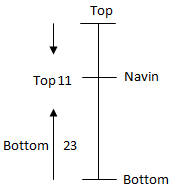Total number of students = 23 + 11 – 1 = 33

Q.2) (c)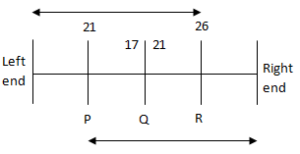Total number of boys = 21 + 17 – 1 = 37

Q.3) (c)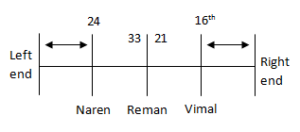Total number of persons = 33 + 21 – 1 = 53

Q.4) (c)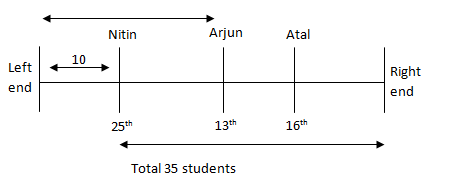Atal third to the right of Arjun

Q.5) (c)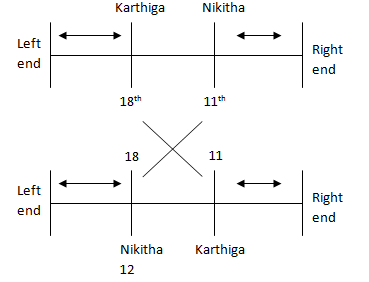Total number of students = 18 + 12 – 1= 29

D.6):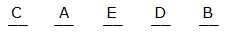(e)

D.7-9):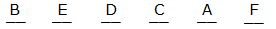Q.7) (d)

D = 33% of (85)

D = 56.66

D = 57%

Q.8) (a)

Q.9) (b)

Q.10) (c)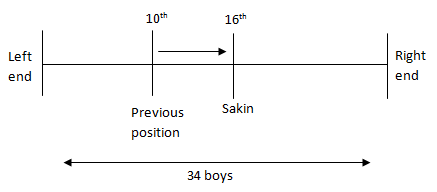Sakin previous position from the right end = 34 – 10 + 1 = 25

Q.11) (b)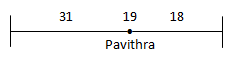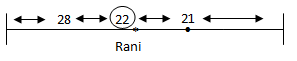Rani position from the left end = 50 – 22 +1 = 29

Q.12) (e)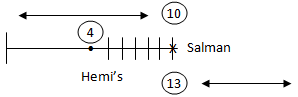Total number of boys in the row = 13+10 -1 = 22

Q.13) (c)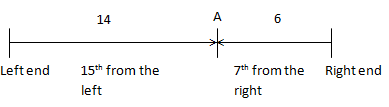14 + 1 + 6 = 21

Q.14) (b)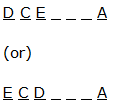Q.15) (d)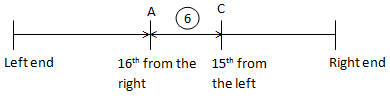9 + 15 – 1 = 23

Q.16) (c)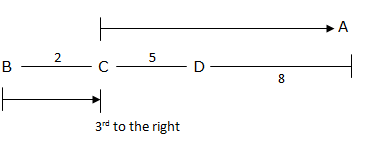Q.17) (d)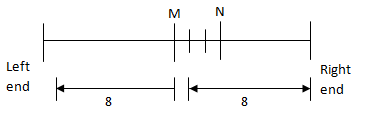(or)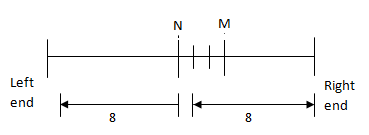D.18-20):

T > Q > G > K > F > L > M

Q.18) (d)

Q.19) (b)

Q.20) (c)

You may also like

SBI CLERK PRELIMS-REASONING DAY-3